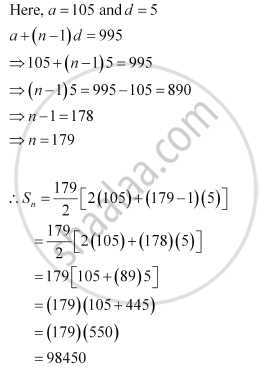# Find the Sum of All Natural Numbers Lying Between 100 and 1000, Which Are Multiples of 5. - Mathematics

Find the sum of all natural numbers lying between 100 and 1000, which are multiples of 5.

#### Solution

The natural numbers lying between 100 and 1000, which are multiples of 5, are 105, 110, … 995.Thus, the sum of all natural numbers lying between 100 and 1000, which are multiples of 5, is 98450.

Concept: Arithmetic Progression (A.P.)
Is there an error in this question or solution?

#### APPEARS IN

NCERT Class 11 Mathematics
Chapter 9 Sequences and Series
Q 2 | Page 185